# Fuchsian equation

(diff) ← Older revision | Latest revision (diff) | Newer revision → (diff)

equation of Fuchsian class

A linear homogeneous ordinary differential equation in the complex domain,(1)

with analytic coefficients, all singular points of which on the Riemann sphere are regular singular points (cf. Regular singular point). Equation (1) belongs to the Fuchsian class if and only if its coefficients have the formwhere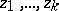are distinct points andis a polynomial of degree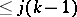. A system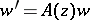ofequations belongs to the Fuchsian class if it has the form(2)

whereare distinct points and the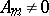are constant-dimensional matrices. The points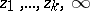are singular for the equation (1) and the system (2). Fuchs' identity holds for (1):where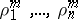are the characteristic exponents at, andthose at(cf. Characteristic exponent). Fuchsian equations (and systems) are also called regular equations (systems). This class of equations and systems was introduced by J.L. Fuchs .

Letbe the Riemann sphere with punctures at the points. Every non-trivial solution of (1) (respectively, every component of a solution of (2)) is an analytic function in. In general, this function is infinite-valued, and all the singular points of (1) (or (2)) are branch points of it of infinite order.

A second-order Fuchsian equation with singular pointshas the form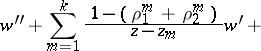(3)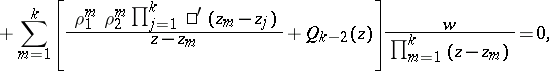where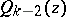is a polynomial of degree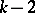. The transformation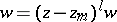takes a Fuchsian equation to a Fuchsian equation, with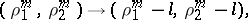and the characteristic exponents at the other singular points are unchanged. By means of such transformations, equation (3) can be reduced to the form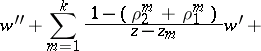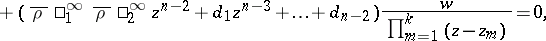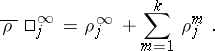A second-order Fuchsian equation withsingular points is completely determined by specifying the values of the characteristic exponents at these points if and only if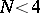. Using a Möbius transformation the equation can be reduced to the form: a)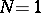,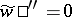; b),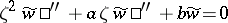(the Euler equation); c)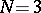— the Papperitz equation (or Riemann equation).

A matrix Fuchsian equation has the form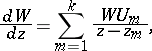(4)

whereare distinct points,is an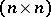-dimensional matrix function, and the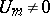are constant matrices. The matrixis called a differential substitution at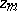. Letbe a closed curve that starts at a non-singular point, is positively oriented and contains only the singular pointinside it. Ifis a solution of (4) that is holomorphic at, then under analytic continuation along,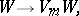(5)

whereis a constant matrix, called an integral substitution at. H. Poincaré (see ) posed the so-called first regular Poincaré problem for a system of the form (4). It consists of the following three problems:

A) to represent the solution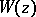in its whole domain of existence;

B) to construct the integral substitutions at the points;

C) to give an analytic characterization of the singularities of the solutions.

In particular, solving problem B) enables one to construct the monodromy group of (4). A solution of the Poincaré problem was obtained by I.A. Lappo-Danilevskii . Let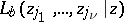,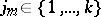,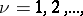be the hyperlogarithms: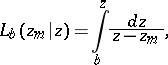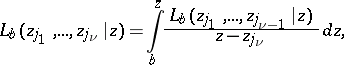let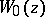be the element (germ) atof a solution of (4), normalized by the condition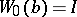, and letbe the analytic function ingenerated by this element. Thenis an entire function of the matrices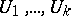and has a series expansionwhich converges uniformly inon every compact set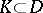. The integral substitution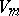atcorresponding to the solutionis an entire function ofand has a series expansion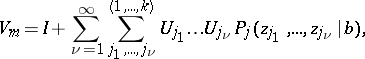where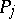can be expressed in terms of hyperlogarithms (see , ).

Formulas that give a solution to problem C) have also been obtained (see ).

How to Cite This Entry:
Fuchsian equation. Encyclopedia of Mathematics. URL: http://encyclopediaofmath.org/index.php?title=Fuchsian_equation&oldid=19254
This article was adapted from an original article by M.V. Fedoryuk (originator), which appeared in Encyclopedia of Mathematics - ISBN 1402006098. See original article